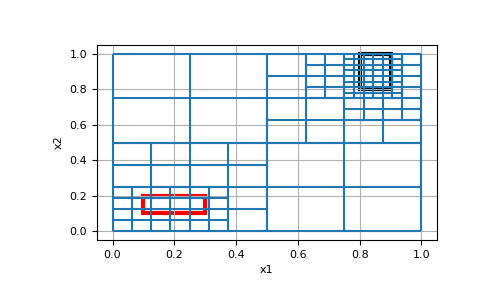# discretize.TreeMesh.refine_box¶

TreeMesh.refine_box(self, x0s, x1s, levels, finalize=True)

Refines the `TreeMesh` within the axis aligned boxes to the desired level

Refines the TreeMesh by determining if a cell intersects the given axis aligned box(es) to the prescribed level(s).

Parameters
x0s(`N`, `dim`) array_like

The minimum location of the boxes

x1s(`N`, `dim`) array_like

The maximum location of the boxes

levels(`N`) array_like `of` `int`

The level to refine intersecting cells to

finalizebool, `optional`

Whether to finalize after refining

Examples

We create a simple mesh and refine the TreeMesh such that all cells that intersect the boxes are at the given levels.

```>>> import discretize
>>> import matplotlib.pyplot as plt
>>> import matplotlib.patches as patches
>>> tree_mesh = discretize.TreeMesh([32, 32])
>>> tree_mesh.max_level
5
```

Next we define the origins and furthest corners of the two rectangles, as well as the level we want to refine them to, and refine the mesh.

```>>> x0s = [[0.1, 0.1], [0.8, 0.8]]
>>> x1s = [[0.3, 0.2], [0.9, 1.0]]
>>> levels = [4, 5]
>>> tree_mesh.refine_box(x0s, x1s, levels)
```

Now lets look at the mesh, and overlay the boxes on it to ensure it refined where we wanted it to.

```>>> ax = tree_mesh.plot_grid()
>>> rect = patches.Rectangle([0.1, 0.1], 0.2, 0.1, facecolor='none', edgecolor='r', linewidth=3)